Get inspired by the success stories of our students in IIT JAM MS, ISI  MStat, CMI MSc DS.  Learn More

# Lock and Key | ISI MStat 2017 PSB | Problem 6This problem is a beautiful and elegant probability based on an elementary problem on how to effectively choose the key to a lock. This gives a simulation environment to problem 6 of ISI MStat 2017 PSB.

## Problem

Suppose you have a 4-digit combination lock, but you have forgotten the correct combination. Consider the following three strategies to find the correct one:
(i) Try the combinations consecutively from 0000 to 9999.
(ii) Try combinations using simple random sampling with replacement from the set of all possible combinations.
(iii) Try combinations using simple random sampling without replacement from the set of all possible combinations.

Assume that the true combination was chosen uniformly at random from all possible combinations. Determine the expected number of attempts needed to find the correct combination in all three cases.

This problem really intrigues me, which gives me the excitement to solve and solve it.

### Prerequisite

• Expectation
• Simple Random Sampling With and Without Replacement
• Discrete Uniform Distribution
• Geometric Distribution
• Smoothing of Expectation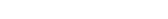. When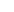and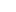have some relationship, this helps us to calculate the expectation.

## Solution

### Consecutive Combination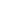~ Discrete Uniform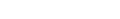Suppose, observe that if you select the keys consecutively, then for the true key, you needattempts. (*)denotes the number of attempts required =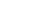due to (*)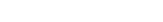.

### Simple Random Sampling With Replacement

This is something no one does, but let's calculate this and see why we don't do this and why we need to remember the keys that don't work like SRSWOR, which is the next case.~ Discrete Uniformdenotes the number of attempts required.Let's say, we have observed, which is fixed and we will calculate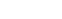.

Observe that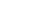~ Geom(\frac{1}{10000}\), since, there are unlimited trials and success occurs if you pick up the right key, which has a probability of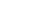.

Therefore,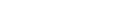. Hence,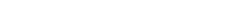#### Let's simulate it.

#Simple Random Sampling with Replacement

NUM = 0
size = 1000 # we have taken 1000 for easier calculation
key = sample(size,1)
number = NULL
random = sample(size,1)
N = 1
for (k in 1:1000) {
number = NULL

for (j in 1:100)
{
while (random != key)
{
random = sample(size,1)
N = N + 1
}
number = c(number,N)
random = sample(size,1)
N = 1
}
NUM = c(NUM,mean(number))
}
mean(NUM)
980.899
hist(NUM)
#Note Replace = TRUE will not work, since, this is an open-ended program

Hence, this is validated by our simulation.

### Simple Random Sampling Without Replacement

This is the sort of key selection, we usually do. Let's investigate it.~ Discrete Uniformdenotes the number of attempts required.Let's say, we have observed, which is fixed and we will calculate.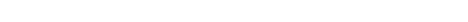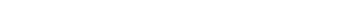. Hence,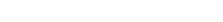.

#Simple Random Sampling without Replacement
average = NULL
number = NULL
size = 10000
key = sample(size,1)
for (j in 1:1000)
{
for (i in 1:100)
{
option = sample(size,size, replace = FALSE)
v = which(option == key)
number = c(number,v)
}
average = c(average,mean(number))
}
mean(average)
4996.567
hist(average, freq = FALSE)

Stay tuned!

Stay Blessed!

This problem is a beautiful and elegant probability based on an elementary problem on how to effectively choose the key to a lock. This gives a simulation environment to problem 6 of ISI MStat 2017 PSB.

## Problem

Suppose you have a 4-digit combination lock, but you have forgotten the correct combination. Consider the following three strategies to find the correct one:
(i) Try the combinations consecutively from 0000 to 9999.
(ii) Try combinations using simple random sampling with replacement from the set of all possible combinations.
(iii) Try combinations using simple random sampling without replacement from the set of all possible combinations.

Assume that the true combination was chosen uniformly at random from all possible combinations. Determine the expected number of attempts needed to find the correct combination in all three cases.

This problem really intrigues me, which gives me the excitement to solve and solve it.

### Prerequisite

• Expectation
• Simple Random Sampling With and Without Replacement
• Discrete Uniform Distribution
• Geometric Distribution
• Smoothing of Expectation. Whenandhave some relationship, this helps us to calculate the expectation.

## Solution

### Consecutive Combination~ Discrete UniformSuppose, observe that if you select the keys consecutively, then for the true key, you needattempts. (*)denotes the number of attempts required =due to (*).

### Simple Random Sampling With Replacement

This is something no one does, but let's calculate this and see why we don't do this and why we need to remember the keys that don't work like SRSWOR, which is the next case.~ Discrete Uniformdenotes the number of attempts required.Let's say, we have observed, which is fixed and we will calculate.

Observe that~ Geom(\frac{1}{10000}\), since, there are unlimited trials and success occurs if you pick up the right key, which has a probability of.

Therefore,. Hence,#### Let's simulate it.

#Simple Random Sampling with Replacement

NUM = 0
size = 1000 # we have taken 1000 for easier calculation
key = sample(size,1)
number = NULL
random = sample(size,1)
N = 1
for (k in 1:1000) {
number = NULL

for (j in 1:100)
{
while (random != key)
{
random = sample(size,1)
N = N + 1
}
number = c(number,N)
random = sample(size,1)
N = 1
}
NUM = c(NUM,mean(number))
}
mean(NUM)
980.899
hist(NUM)
#Note Replace = TRUE will not work, since, this is an open-ended program

Hence, this is validated by our simulation.

### Simple Random Sampling Without Replacement

This is the sort of key selection, we usually do. Let's investigate it.~ Discrete Uniformdenotes the number of attempts required.Let's say, we have observed, which is fixed and we will calculate.. Hence,.

#Simple Random Sampling without Replacement
average = NULL
number = NULL
size = 10000
key = sample(size,1)
for (j in 1:1000)
{
for (i in 1:100)
{
option = sample(size,size, replace = FALSE)
v = which(option == key)
number = c(number,v)
}
average = c(average,mean(number))
}
mean(average)
4996.567
hist(average, freq = FALSE)

Stay tuned!

Stay Blessed!

This site uses Akismet to reduce spam. Learn how your comment data is processed.

### 2 comments on “Lock and Key | ISI MStat 2017 PSB | Problem 6”

1.critic's bug says:

the expected value in uniform should be 5000.

2.SPORTS STORY says:

It is written that N= U +1. But after that , it is written E(N) = E( U ) ? Isn't should be "E(N) = E( U ) +1"?

### Knowledge Partner